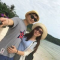Class Central is learner-supported. When you buy through links on our site, we may earn an affiliate commission.

# Introduction to Statistics

### Overview

This course is designed to explain the fundamental of statistics. The course contains four weeks or four modules. The first module is devoted to the main concepts of statistics and data analysis. First of all we will introduce the concepts of sample, general population, descriptive statistics and normal distribution. At the end of the first module we will discuss the idea of statistical inference, one of the most important topics of our course. If you have just started to study statistics look more closely at the first week. All the lessons of the first module are extremely important to enable you to understand the rest of the course and more complicated concepts and methods of statistics. Each module contains lessons with short theoretical video lectures mixed with practical problems.

### Syllabus

Introduction to Hypothesis Testing

1.1 Introduction

1.2 Sample and Population

1.3 Types of Variables in Statistics

1.4 Descriptive Statistics (Central Tendency Measures)

1.5 Descriptive Statistics (Variance and Quantiles)

1.6 Normal Distribution

1.7 Central Limit Theorem

1.8 Confidence Intervals

1.9 Statistical Hypothesis Testing

Categorical Data Analysis

2.1 Introduction. Categorical Data in Statistics

2.2 Chi-Squared Distance

2.3 Pearson Distribution, Degrees of Freedom and Chi-Squared Test

2.4 Contingency Table Analysis

2.5 P-value Calculation

2.6 Fisher's Exact Test

T- test and Analysis of Variance

3.1 Student's t-Distribution

3.2 Student's t-Test

3.3 One-Way ANOVA

3.4 Post Hoc Analysis

Correlation and Linear Regression

4.1 Definitions of Covariance and Correlation

4.2 Simple Linear Regression. Least Squares. Residuals.

4.3 Predictions from Linear Regression. Assumptions.

4.4 Multiple Linear Regression

### Taught by

Anatoliy Karpov and Polina Drozdova

## Reviews

5.0 rating, based on 1 Class Central review

Start your review of Introduction to Statistics

•Nguyen Thuy Giang

Nguyen Thuy Giang is taking this course right now, spending 4 hours a week on it and found the course difficulty to be medium.

It helps me to understand the fundamental of statistics in the easiest way. It provides specific examples for each concepts and problems. Very good!

### Never Stop Learning.

Get personalized course recommendations, track subjects and courses with reminders, and more.